# Forestry: Optimized Required Logging Using Systems of Equations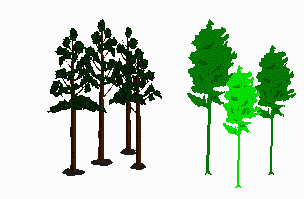My logging company has a contract with a local mill to provide 1000 m³ of Lodgepole pine, 800 m³ of spruce, and 600 m³ of Douglas fir logs per month. I have three regions available to me for logging. The following table gives the species mix, and timber density for each region.

Region Volume/hectare % Pine % Spruce % Fir
West 330 m³ / ha 70 % 20 % 10 %
North 390 m³ / ha 10 % 60 % 30 %
East 290 m³ / ha 5 % 20 % 75 %

How many hectares should I log in each operating region listed above to deliver exactly the required volume of logs? I don’t want to have to store logs so I don’t want any left over at the end of each month, but I do need to make my quota.

## Solution:

Problems such as this can be solved using simultaneous equations by identifying quantities we need to know and then finding relationships between them.

### Setting up the problem - translating from words to symbols:

1. Since we want to know the number of hectares that should be logged in each region, start by letting
• x = number of hectares logged in West region
• y = number of hectares logged in North region
• z= number of hectares logged in East region
2. The total volume of wood logged in West region will be the volume of logs / hectare times the number of hectares, or (330 m3/ha)(xha) = 330xm3.
3. The total volume of Lodgepole pine logged in the West region is the total volume of wood times the percentage of pine. Since 70% = 0.7, the total amount of pine from the West region is 0.7(330x)m3.
4. If we follow the same steps as 2 and 3 for the North region, we get the total volume of Lodgepole pine logged in the North region is 0.1(390y)m3.
5. Doing the same for the East region, we get 0.05(290z)m3.
6. Steps 3, 4, and 5 calculate the volume of Lodgepole pine from the three regions. We know the total lodgepole pine needed is 1000 m3. So we must have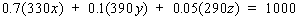7. If the same steps are followed for spruce and Douglas fir, two more equations will be obtained. These will be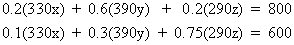### Solving a set of simultaneous equations:

Each equation above contains three unknowns. Let us repeat these equations and simplify by multiplying out the numbers: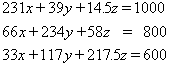There are many ways to solve for the unknown values x, y and z. We will consider one way. This is called elimination. We take pairs of equations and eliminate one of the variables.

1. Solve each of the first two equations for z and equate them. The result of this procedure is: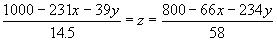2. Solve each of the first and last equations for z and equate them.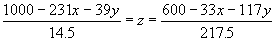3. Remove the z and simplify the equations in 1 and 2.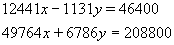We have reduced the problem from one of solving 3 equations with 3 unknowns to one of solving 2 equations in 2 unknowns
4. Let us solve each of the equations in step 3 for y and equate them.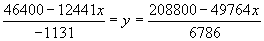5. Remove the y and simplify the equation to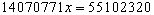6. Solve for x. We get x = 3.92
7. Solve for y using one of the equations in step 4. We get y = 2.05
8. Solve for z using any one of the equations in steps 1 or 2. We get z = 1.06